StatLect

R squared of a linear regression

How good is a linear regression model in predicting the output variable on the basis of the input variables? How much of the variability in the output is explained by the variability in the inputs of a linear regression? The R squared of a linear regression is a statistic that provides a quantitative answer to these questions.Definition

Before giving a definition of the R squared of a linear regression, we warn our readers that several slightly different definitions can be found in the literature, and that usually these definitions are equivalent only in the special, but important case in which the linear regression includes a constant among its regressors. We choose the definition given here because we think it is easier to understand, but our readers are invited to consult also other sources.

Consider the linear regression modelwhereis avector of inputs andis avector of regression coefficients. Suppose that we have a sample ofobservations, for. Given an estimateof(for example, an OLS estimate), we can compute the residuals of the regression:Denote bythe unadjusted sample variance of the outputs: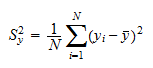whereis the sample meanThe sample varianceis a measure of the variability of the outputs, that is, of the variability that we are trying to explain with the regression model.

Denote bythe mean of squared residuals: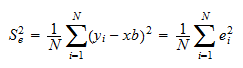which coincides with the unadjusted sample variance of the residuals when the sample mean of the residuals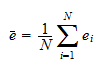is equal to zero. Unless stated otherwise, we are going to maintain the assumption thatin what follows.

The sample varianceis a measure of the variability of the residuals, that is, of the part of the variability of the outputs that we are not able to explain with the regression model. Intuitively, when the predictions of the linear regression model are perfect, then the residuals are always equal to zero and their sample variance is also equal to zero. On the contrary, the less the predictions of the linear regression model are accurate, the highest is the variance of the residuals.

We are now ready to give a definition of R squared.

Definition The R squared of the linear regression, denoted by, iswhereis the sample variance of the residuals andis the sample variance of the outputs.

Thus, the R squared is a decreasing function of the sample variance of the residuals: the higher the sample variance of the residuals is, the smaller the R squared is.

Note that the R squared cannot be larger than 1: it is equal to 1 when the sample variance of the residuals is zero, and it is smaller than 1 when the sample variance of the residuals is strictly positive.

The R squared is equal to 0 when the variance of the residuals is equal to the variance of the outputs, that is, when predicting the outputs with the regression model is no better than using the sample mean of the outputs as a prediction.

It is possible to prove that the R squared cannot be smaller than 0 if the regression includes a constant among its regressors andis the OLS estimate of(in this case we also have that). Outside this important special case, the R squared can take negative values.

In summary, the R square is a measure of how well the linear regression fits the data (in more technical terms, it is a goodness-of-fit measure): when it is equal to 1 (and), it indicates that the fit of the regression is perfect; and the smaller it is, the worse the fit of the regression is.

The adjusted R squared is obtained by using the adjusted sample variances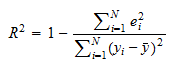and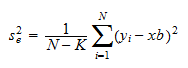instead of the unadjusted sample variancesand.

This is done becauseandare unbiased estimators ofandunder certain assumptions (see the lectures entitled Variance estimation and The Normal Linear Regression Model).

Definition The adjusted R squared of the linear regression, denoted by, iswhereis the adjusted sample variance of the residuals andis the adjusted sample variance of the outputs.

The adjusted R squared can also be written as a function of the unadjusted sample variances:Proof

This is an immediate consequence of the fact that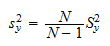and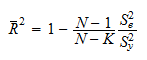The ratioused in the formula above is often called a "degrees of freedom adjustment".

The intuition of the adjustment is as follows. When the numberof regressors (and regression coefficients) is large, then the R squared tends to be small because the mere fact of being able to adjust many regression coefficients allows to significantly reduce the variance of the residuals (a phenomenon known as over-fitting; the extreme case is when the number of regressorsis equal to the number of observationsand we can chooseso as to make all the residuals equal to). But being able to mechanically make the variance of the residuals small by adjustingdoes not mean that the variance of the errors of the regressionis as small. The degrees of freedom adjustment allows to take this fact into consideration and to avoid under-estimating the variance of the error terms.

In more thechnical terms, the idea behind the adjustment is that what we would really like to know is the quantitybut the unadjusted sample variancesandare biased estimators ofand(the bias is downwards, that is, they tend to underestimate). As a consequence, we estimateandwith the adjusted sample variancesand, which are unbiased estimators.

The book

Most of the learning materials found on this website are now available in a traditional textbook format.

Glossary entries
Share